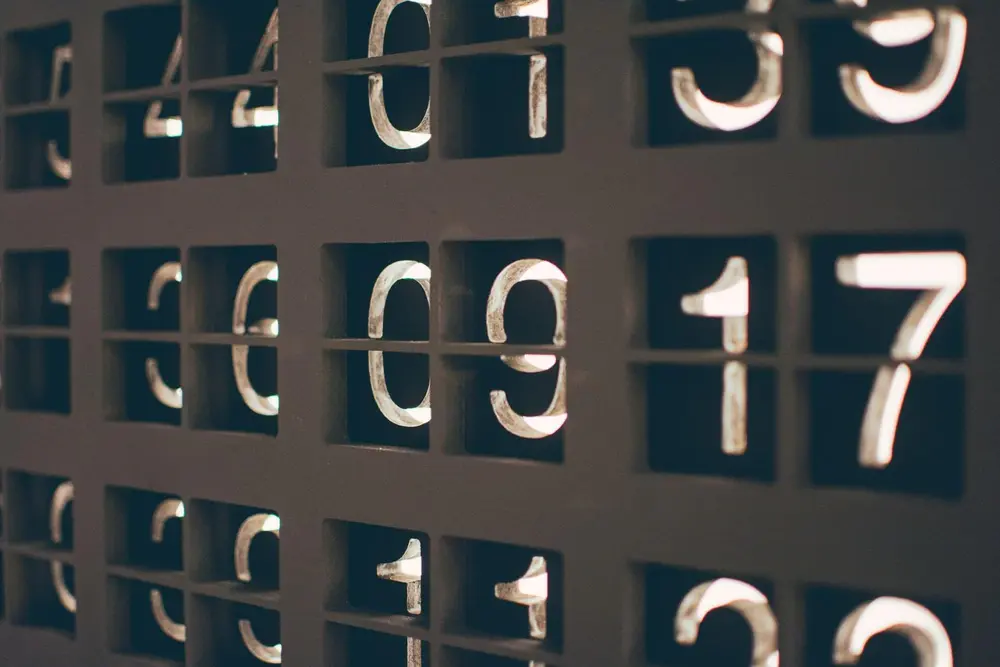Think of two small numbers __ __ Put them inside this problem: (x + __ ) (x + __ )

and multiply them out so it looks like this: x2 + __ x + __

In the form ax2 + bx + c we can say that: a = 1, b = __ and c = __

Now put the numbers a, b and c into this formula

This gives two answers: x = __ and x = __

Do they correspond to the numbers you first thought of?

Yes? Well done!

Number

Number

Number

Number

Number

Number